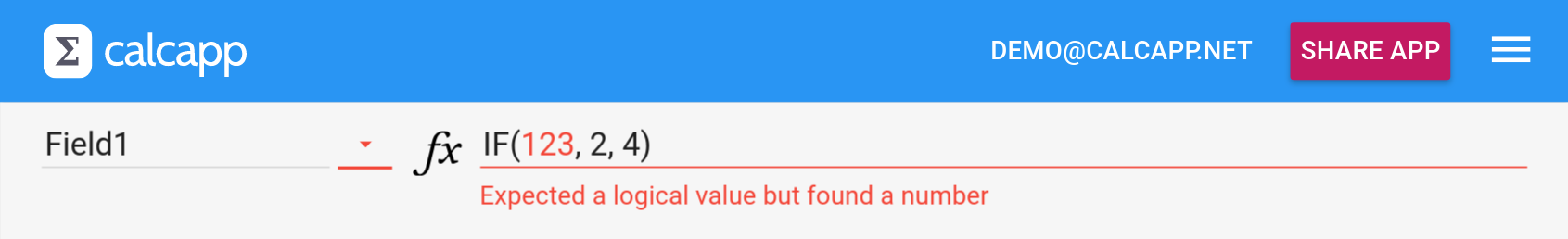• Learn
• Blog
• Pricing

# Feature: The new TONUMBER, TOLOGICAL and TOTEXT functions

The new formula functions TONUMBER, TOLOGICAL and TOTEXT make it easy to convert values to numbers, logical values and text strings, respectively. Converting numbers stored as text to true numbers was previously impossible if the number had a fractional part.

Like spreadsheets, Calcapp supports three types of values: numbers, logical values (TRUE or FALSE) and text strings. Unlike spreadsheets, Calcapp is very particular about these types. If it spots what appears to be an error, it displays an error message, asking you to correct your formula.

For instance, the first parameter to the IF formula function is a condition determining whether the second parameter or the third parameter should be used. As such, it is a logical value, that is, a value which evaluates to either TRUE or FALSE. `Field2 > 2` fits the bill, but `123` does not:A spreadsheet, on the other hand, would happily allow this formula without showing an error message. It would interpret 123 as TRUE (for a number, only zero is interpreted as FALSE). Essentially, when spreadsheets encounter values which are of the wrong type, they often don’t present an error but instead silently convert the value to the desired type. (Technically, this process is called type coercion.)

Calcapp doesn’t work that way and insists that you use a value of the proper type. If a logical value is called for, only a logical value is accepted. (The technical term for this design choice is called static typing.)

Most of the time, static typing helps you save time. If you get the parameter order wrong and the types don’t match, you’ll get an error message, saving you from trying to understand why your app presents incorrect results. Some of the time, though, it gets in the way, making it harder to get your formulas accepted.

Our next release improves on this aspect by making it more straight-forward to explicitly tell Calcapp to convert a value from one type to another. The new functions are named TONUMBER, TOLOGICAL and TOTEXT and do what their names imply: TONUMBER takes any value and converts it to a number, TOLOGICAL converts any value to a logical value and TOTEXT converts any value to a text string.

These new functions are specific to Calcapp and join a small number of Calcapp-specific functions (which are all collected in the Calcapp category of the reference sidebar). Apart from the new functions, only ISDEFINED and BLANK and the color functions are specific to Calcapp.

We avoid adding functions specific to Calcapp and instead try to rely only on the functions which are more or less universally supported across different spreadsheets. That makes is easy not just to copy formulas from spreadsheets to Calcapp, but to go in the other direction as well, if needs warrant. We think that including these three new Calcapp-specific functions is warranted, as Calcapp doesn’t do type conversion automatically, unlike spreadsheets.

Earlier, converting values to different types was at best unintuitive and at worst impossible. The CONCATENATE function accepts any number of parameters (where said parameters can be of any type) and joins them together. If invoked with only one parameter, it could actually be used as a means of converting any value to a text string, but the name was unfortunate. Worse, the DECIMAL function can be used to convert text strings representing numbers to true numbers, but would silently discard the fractional part. The new TOTEXT and TONUMBER functions fix these problems. (The TOLOGICAL function is mostly provided for completeness’ sake.)

When TOTEXT converts numbers to text strings and when TONUMBER converts text strings to numbers, said text strings use a decimal point as opposed to a decimal comma, a leading minus symbol instead of a parentheses-enclosed number and thousands separators are not used. Functions capable of working with regionally-formatted numbers may be added in the future. (March, 2018, update: And here they are!) For detailed information on how the new functions behave, refer to the reference sidebar.

Also, we have made two additional formula-related changes. Both changes improve compatibility with spradsheets, increasing the chances that you can copy spreadsheet formulas directly to Calcapp Creator:

• The IF formula function now accepts only two parameters in addition to the standard three. When invoked with two parameters, the third parameter is assumed to be a blank value. In other words, `IF(Field1 > 2, 42)` is equivalent to `IF(Field1 > 2, 42, BLANK())`. A blank value is treated as the number zero, the value FALSE or the empty text string depending on context. That means that `IF(FALSE, 1) + 4` is equivalent to ```IF(FALSE, 1, BLANK()) + 4```, which in this context is equivalent to `IF(FALSE, 1, 0) + 4` and as a result evaluates to 4 (0 + 4).

• When you’re writing number constants in formulas that are less than one but greater than zero, such as `0.42`, you can now leave out the leading zero. As a result, `.42` and `0.42` are now equivalent.